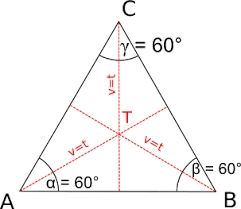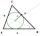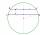# Equilateral triangle vs circle

Find the area of an equilateral triangle inscribed in a circle of radius r = 9 cm. What percentage of the circle area does it occupy?

S =  105.2221 cm2
p =  41.3497 %

### Step-by-step explanation:Did you find an error or inaccuracy? Feel free to write us. Thank you!Tips to related online calculators
Pythagorean theorem is the base for the right triangle calculator.

#### You need to know the following knowledge to solve this word math problem:

We encourage you to watch this tutorial video on this math problem:

## Related math problems and questions:

• Inscribed circleXYZ is right triangle with right angle at the vertex X that has inscribed circle with a radius 5 cm. Determine area of the triangle XYZ if XZ = 14 cm.
• An equilateralAn equilateral triangle is inscribed in a square of side 1 unit long so that it has one common vertex with the square. What is the area of the inscribed triangle?
• Cathethus and the inscribed circleIn a right triangle is given one cathethus long 14 cm and the radius of the inscribed circle of 5 cm. Calculate the area of this right triangle.
• PentagonCalculate the length of side, circumference and area of a regular pentagon, which is inscribed in a circle with radius r = 6 cm.
• Equilateral triangleCalculate the area of an equilateral triangle with circumference 72cm.
• Inscribed circleThe circle inscribed in a triangle has a radius of 3 cm. Express the area of the triangle using a, b, c.
• Right triangleA circle with a radius of 5 cm is described in a right triangle with a 6 cm leg. What is the height at the hypotenuse of this triangle?
• Equilateral triangleThe equilateral triangle has a 23 cm long side. Calculate its content area.
• Ratio of sidesCalculate the area of a circle with the same circumference as the circumference of the rectangle inscribed with a circle with a radius of r 9 cm so that its sides are in ratio 2 to 7.
• Circle describedThe radius of the circle described to the right triangle with 6 cm long leg is 5 cm. Calculate the circumference of this triangle.
• 30-gonAt a regular 30-gon the radius of the inscribed circle is 15cm. Find the "a" side size, circle radius "R", circumference, and content area.
• Hexagonal pyramidCalculate the surface area of a regular hexagonal pyramid with a base inscribed in a circle with a radius of 8 cm and a height of 20 cm.
• Quarter circleWhat is the radius of a circle inscribed in the quarter circle with a radius of 100 cm?
• Two chordsIn a circle with a radius of 8.5 cm, two parallel chords are constructed, the lengths of which are 9 cm and 12 cm. Find the distance of the chords in a circle.
• Equilateral triangleA square is inscribed into an equilateral triangle with a side of 10 cm. Calculate the length of the square side.
• RT sidesFind the sides of a rectangular triangle if legs a + b = 17cm and the radius of the written circle ρ = 2cm.
• 9-gon pyramidCalculate the volume and the surface of a nine-sided pyramid, the base of which can be inscribed with a circle with radius ρ = 7.2 cm and whose side edge s = 10.9 cm.